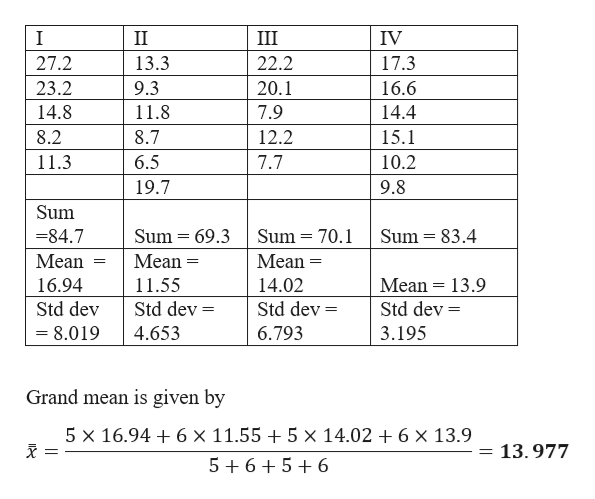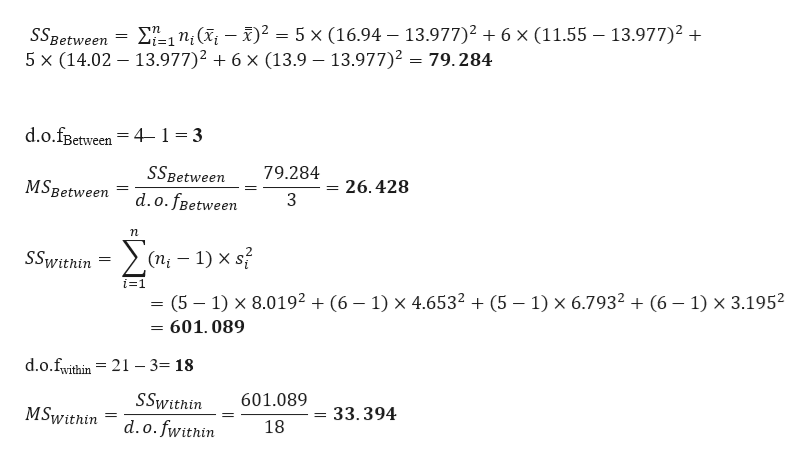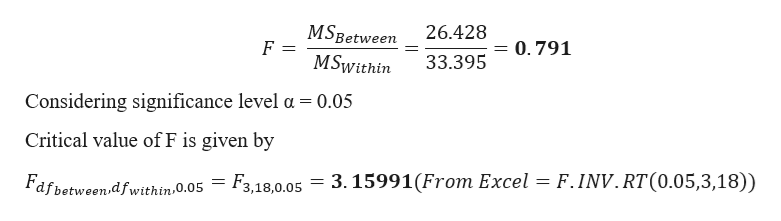# How productive are U.S. workers? One way to answer this question is to study annual profits per employee. A random sample of companies in computers (I), aerospace (II), heavy equipment (III), and broadcasting (IV) gave the following data regarding annual profits per employee (units in thousands of dollars).IIIIIIIV27.213.322.217.323.29.320.116.614.811.87.914.48.28.712.215.111.36.57.710.2 19.7 9.8Shall we reject or not reject the claim that there is no difference in population mean annual profits per employee in each of the four types of companies? Use a 5% level of significance.(b) Find SSTOT, SSBET, and SSW and check that SSTOT = SSBET + SSW. (Use 3 decimal places.)SSTOT= SSBET= SSW= Find d.f.BET, d.f.W, MSBET, and MSW. (Use 3 decimal places for MSBET, and MSW.)dfBET= dfW= MSBET= MSW= (c) Find the value of the sample F statistic. (Use 3 decimal places.)(d) Make a summary table for your ANOVA test.

Question
1 views

How productive are U.S. workers? One way to answer this question is to study annual profits per employee. A random sample of companies in computers (I), aerospace (II), heavy equipment (III), and broadcasting (IV) gave the following data regarding annual profits per employee (units in thousands of dollars).

 I II III IV 27.2 13.3 22.2 17.3 23.2 9.3 20.1 16.6 14.8 11.8 7.9 14.4 8.2 8.7 12.2 15.1 11.3 6.5 7.7 10.2 19.7 9.8

Shall we reject or not reject the claim that there is no difference in population mean annual profits per employee in each of the four types of companies? Use a 5% level of significance.

(b) Find SSTOT, SSBET, and SSW and check that SSTOT = SSBET + SSW. (Use 3 decimal places.)

 SSTOT = SSBET = SSW =

Find d.f.BET, d.f.W, MSBET, and MSW. (Use 3 decimal places for MSBET, and MSW.)

 dfBET = dfW = MSBET = MSW =

(c) Find the value of the sample F statistic. (Use 3 decimal places.)

(d) Make a summary table for your ANOVA test.

check_circle

Step 1

Given data and calculation is shown belowhelp_outlineImage TranscriptioncloseII Ш IV 27.2 13.3 22.2 17.3 23.2 9.3 20.1 16.6 14.8 7.9 11.8 14.4 15.1 8.2 8.7 12.2 11.3 6.5 7.7 10.2 19.7 9.8 Sum =84.7 Sum = 69.3 Sum = 70.1 Sum = 83.4 Mean Mean = Mean = %3D 16.94 11.55 14.02 Mean = 13.9 Std dev Std dev = Std dev = Std dev = = 8.019 4.653 6.793 3.195 Grand mean is given by 5 x 16.94 + 6 × 11.55 + 5 × 14.02 + 6 × 13.9 I = 13.977 5+6+5+ 6 fullscreen
Step 2

SSBetween is given byhelp_outlineImage TranscriptioncloseSSBetween = E=1n;(X¡ – X)² = 5 × (16.94 – 13.977)² + 6 × (11.55 – 13.977)² + 5 x (14.02 – 13.977)² + 6 x (13.9 – 13.977)2 = 79.284 d.o.fßetween = 4-1= 3 SSBetween 79.284 MSBetween = 26, 428 d.o. fBetween 3 (п, — 1) x s? SSwithin i=1 (5 – 1) × 8.019² + (6 – 1) × 4.6532 + (5 – 1) × 6.7932 + (6 – 1) × 3.1952 601.089 d.o.fwithin = 21 – 3= 18 SSwithin 601.089 MSwithin = 33.394 d.o.fwithin 18 fullscreen
Step 3

Test-statistic is...help_outlineImage TranscriptioncloseMSBetween MSwithin 26.428 0.791 33.395 Considering significance level a = 0.05 Critical value of F is given by Fafbetween.df within.0.05 F3,18,0.05 = 3. 15991(From Excel = F.INV.RT(0.05,3,18)) fullscreen

### Want to see the full answer?

See Solution

#### Want to see this answer and more?

Solutions are written by subject experts who are available 24/7. Questions are typically answered within 1 hour.*

See Solution
*Response times may vary by subject and question.
Tagged in

### Hypothesis Testing